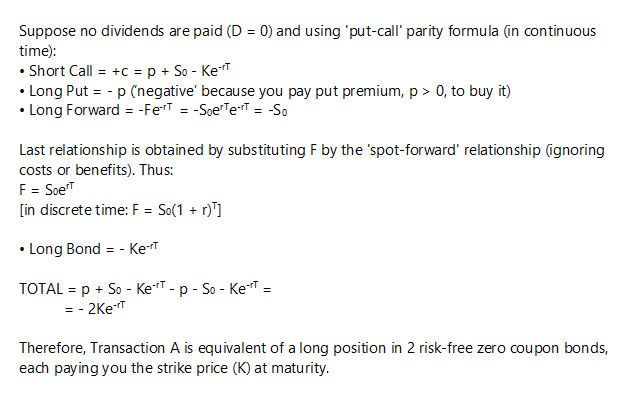# Doubt for Put Call Forward parity

Can someone explain this question to me? I tried rearranging the Put-Call-Forward Parity and got B (C-P = F/(1+rf)T - X/(1+rf)T) , but A is the correct answer.

Q. Under put–call–forward parity, which of the following transactions is risk free?

A. Short call, long put, long forward contract, long risk-free bond

B. Long call, short put, long forward contract, short risk-free bond

C. Long call, long put, short forward contract, short risk-free bond

Can someone please explain me the answer for this question, I tried to understand the same from 2-3 CFA aspirates, but honestly they are not able to explain me the reasoning. I would be thankful if someone can please help me in understanding the concept behind this.

Thanks

First, it’s a weird question.

In essence, they’re (first) asking you to solve the put-call forward parity equation for . . . wait for it! . . . zero!

Note that zero is risk-free.

P_0\ + \frac{F_T}{\left(1 + r_f\right)^T} = C_0\ + \frac{X}{\left(1 + r_f\right)^T}
P_0\ - C_0\ + \frac{F_T}{\left(1 + r_f\right)^T} - \frac{X}{\left(1 + r_f\right)^T} = 0

Note that the put and the forward have the same sign: you have to be long both or else short both. So B can’t possibly be correct, nor can C.

But how did they get to A? My equation has a short bond, not a long bond.

Here’s the subtle part: if you add a risk-free asset to a risk-free position, the position remains risk-free.

Instead of adding a long bond to my equation, I’ll add two: one to cancel my short bond and one to give me a long bond:

P_0\ - C_0\ + \frac{F_T}{\left(1 + r_f\right)^T} + \frac{X}{\left(1 + r_f\right)^T} = 2\frac{X}{\left(1 + r_f\right)^T}

So transaction A is equivalent to buying two risk-free bonds.

More than a little sneaky. (However, as it’s easy to eliminate B and C, maybe sneaky, but easy.)

thank you so much s2m! you looks like have passed CFA LEVEL 10000.

And just without the accurate mathematical explanation s2000 provided above, you can see that an answer with “long call” in it is most likely incorrect. Because you need to move the call to the other side of the equation to isolate for the risk free zero coupon bond equal to the present value of the strike price (I just call it ‘B’ in my mental lexicon). So it should be a short call in the correct answer. I would approach such questions in this manner, just set up the equation and switch things left or right as required to isolate for element they are asking for. The risk-free bond and the call are on the same side of the equation. So isolating for one of them means the other needs to be switched to the other side of the equation with a negative sign change. Cheers - good luck - you got this👍